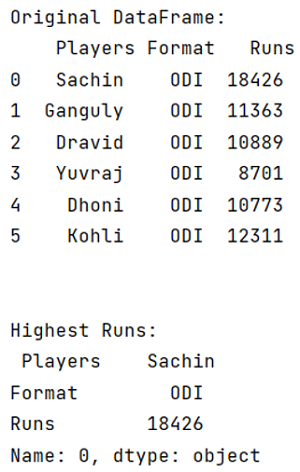# How to find row where values for column is maximal in a Pandas DataFrame?

Given a Pandas DataFrame, we have to find row where values for column is maximal. By Pranit Sharma Last updated : September 20, 2023

Columns are the different fields that contain their particular values when we create a DataFrame. We can perform certain operations on both rows & column values. Rows in pandas are the different cell (column) values that are aligned horizontally and also provide uniformity. Each row can have the same or different value. Rows are generally marked with the index number but in pandas, we can also assign index names according to the needs. Here, we are going to find the row whose column has the maximum value.

## Problem statement

Given a Pandas DataFrame, we have to find row where values for column is maximal.

## Finding row where values for column is maximal in a Pandas DataFrame

For this purpose, pandas provide idx.max() method, which will return that index where the column value is max. Then with the help of pandas.DataFrame.loc property, we will get the row by passing the index.

Syntax:

```DataFrame.idxmax(
axis=0,
skipna=True
)
```

Parameter(s): It takes two parameters, axis and skipna which are usually defined to check axis and to skip the Null values respectively.

Note

To work with pandas, we need to import pandas package first, below is the syntax:

```import pandas as pd
```

Let us understand with the help of an example.

## Python program to find row where values for column is maximal in a Pandas DataFrame

```# Importing pandas package
import pandas as pd

# creating a dictionary
d = {
"Players":['Sachin','Ganguly','Dravid','Yuvraj','Dhoni','Kohli'],
"Format":['ODI','ODI','ODI','ODI','ODI','ODI'],
"Runs":[18426,11363,10889,8701,10773,12311]
}

# Now we will create DataFrame
df = pd.DataFrame(d)

# Viewing the DataFrame
print("Original DataFrame:\n",df,"\n\n")

# Find max Runs
result = df['Runs'].idxmax()

# Using df.loc
row = df.loc[result]

# Print row
print("Highest Runs:\n",row)
```

### Output

The output of the above program is: